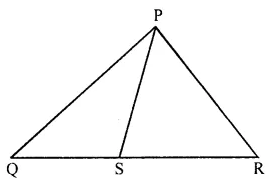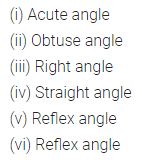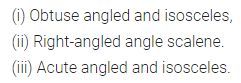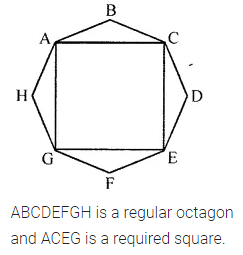ML Aggarwal Class 6 Solutions Chapter 11 Understanding Symmetrical Shapes Check Your Progress for ICSE Understanding Mathematics acts as the best resource during your learning and helps you score well in your exams.

## ML Aggarwal Class 6 Solutions for ICSE Maths Chapter 11 Understanding Symmetrical Shapes Check Your Progress

Question 1.
In the given figure, identify the longest and shortest line segments by measuring their lengths.Solution:Question 2.
Where will the hour hand of a clock stop if it starts from 10 and turns through 3 right angles?
Solution:
7

Question 3.
Classify the angles whose measures are given below:
(i) 56°
(ii) 125°
(iii) 90°
(iv) 180°
(v) 215°
(vi) 328°
Solution:Question 4.
Name the types of the following triangles:
(i) ∆ABC with AB = 8 cm, AC = 7 cm and BC = 5.5 cm.
(ii) ∆PQR with PQ = PR = 5 cm and QR = 7.3 cm.
(iii) ∆DEF with D = ∠90°.
(iv) ∆XYZ with ∠Y = 90° and XY = YZ.
(v) ∆LMN with ∠L = 30°, ∠M = 70° and ∠N = 80°.
Solution:Question 5.
Name each of the following triangles in two different ways (you may use ruler and protractor):Solution:Question 6.
State whether the following statements are true or false:
(i) A rectangle is a regular quadrilateral.
(ii) A rhombus is a regular quadrilateral.
(iii) Every parallelogram is a rhombus.
(iv) The diagonals of a rhombus intersect at right angles.
(v) A polygon having 6 sides is called an octagon.
(vi) A road roller has two plane circular faces and one curved face.
(vii) A rectangular pyramid has 5 rectangular faces.
Solution:Question 7.
Draw a rough sketch of a regular octagon and draw a square by joining exactly four of its vertices.
Solution: